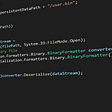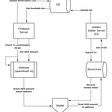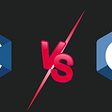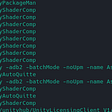# Analysis report

• 48 first-level warnings;
• 79 second-level warnings;
• 261 third-level warnings.
• 124 first-level warnings;
• 272 second-level warnings;
• 787 third-level warnings (some of them are pretty interesting too).

# Diagnostic messages

`bool dgCollisionConvexHull::Create (dgInt32 count,....){  ....  dgStack<dgVector> tmp(3 * count);  for (dgInt32 i = 0; i < count; i ++)   {    tmp[i] = dgFloat32 (buffer[i*3 + 0]);    tmp[i] = dgFloat32 (buffer[i*3 + 1]);    tmp[i] = dgFloat32 (buffer[i*3 + 2]);    tmp[i] = dgFloat32 (0.0f);  }  ....}`
`void dgBody::AddBuoyancyForce (....){  ....  damp = (m_omega % m_omega) * dgFloat32 (10.0f) *        fluidAngularViscousity;   damp = GetMax (GetMin ((m_omega % m_omega) *        dgFloat32 (1000.0f) *        fluidAngularViscousity, dgFloat32(0.25f)),        dgFloat32(2.0f));  ....}`
`void MultibodyBodyCar(DemoEntityManager* const scene){  ....  int count = 10;  count = 0;  for (int i = 0; i < count; i++)   {    for (int j = 0; j < count; j++)     {      dMatrix offset(location);      offset.m_posit += dVector (j * 5.0f + 4.0f, 0.0f, i * 5.0f, 0.0f);      //manager->CreateSportCar(offset, viperModel.GetData());      manager->CreateOffRoadCar(offset, monsterTruck.GetData());    }  }  ....}`
`void dString::LoadFile (FILE* const file){  ....  size_t ret = fread(m_string, 1, size, file);  ret = 0;  ....}`
`void DemoEntityManager::DeserializeFile (....){  ....  size_t ret = fread(buffer, size, 1, (FILE*) serializeHandle);  ret = 0;  ....}`
`bool dCholeskyWithRegularizer(....){  ....  int count = 0;  while (!pass && (count < 10))  {    ....  }  ....}`
`void dgPolyhedra::Triangulate (....){  ....  ptr = edge;  ....  while (ptr != edge);  ....}`
`StupidComplexOfConvexShapes (...., int count){  count = 40;  //count = 1;  ....}`
`void UpdateDriverInput(dVehicle* const vehicle, dFloat timestep) {  ....  int axisCount = scene->GetJoystickAxis(axis);  axisCount = 0;  if (axisCount)  {    ....  }  ....}`
`GLFWvidmode* _glfwPlatformGetVideoModes(_GLFWmonitor* monitor, int* count){  GLFWvidmode* result = NULL;  ....  for (i = 0;  i < *count;  i++)    {    if (_glfwCompareVideoModes(result + i, &mode) == 0)      break;    }}`
`void dgMeshEffect::EndBuildFace (){  ....  if (m_attrib.m_binormalChannel.m_count) <=  {    attibutes.m_binormalChannel.      PushBack(m_attrib.m_binormalChannel[m_constructionIndex + i]);  }  if (m_attrib.m_binormalChannel.m_count) <=   {    attibutes.m_colorChannel.      PushBack(m_attrib.m_colorChannel[m_constructionIndex + i]);  }}`
`if (m_attrib.m_colorChannel.m_count) <= {  attibutes.m_colorChannel.  PushBack(m_attrib.m_colorChannel[m_constructionIndex + i]);}`
`void dCustomDoubleHingeActuator::EnabledAxis0(bool state){  m_axis0Enable = state;  <=}void dCustomDoubleHingeActuator::EnabledAxis1(bool state){  m_axis0Enable = state;  <=}`
`void dCustomDoubleHingeActuator::EnabledAxis1(bool state){  m_axis1Enable = state;}`
`WoodVoronoidEffect(....){  ....  for (int i = 0; i < count; i ++)   {    dFloat x = dGaussianRandom(size.m_x * 0.1f);    dFloat y = dGaussianRandom(size.m_y * 0.1f);  <=    dFloat z = dGaussianRandom(size.m_y * 0.1f);  <=  ....  }  ....}`
`dFloat z = dGaussianRandom(size.m_z * 0.1f);`
`DG_INLINE dgMinkFace* dgContactSolver::AddFace(dgInt32 v0,dgInt32 v1,                                               dgInt32 v2){  dgMinkFace* const face = NewFace();  face->m_mark = 0;   ....}`
`DG_INLINE dgMinkFace* dgContactSolver::NewFace(){  dgMinkFace* face = (dgMinkFace*)m_freeFace;  if (m_freeFace)   {    m_freeFace = m_freeFace->m_next;  } else   {    face = &m_facePool[m_faceIndex];    m_faceIndex++;    if (m_faceIndex >= DG_CONVEX_MINK_MAX_FACES)     {      return NULL;    }  }#ifdef _DEBUG    memset(face, 0, sizeof (dgMinkFace));#endif  return face;}`
`bool dgPolyhedra::PolygonizeFace(....){  ....  dgEdge* const perimeter = flatFace.AddHalfEdge                           (edge1->m_next->m_incidentVertex,                            edge1->m_incidentVertex);  perimeter->m_twin = edge1;  ....}`
`dgEdge* dgPolyhedra::AddHalfEdge (dgInt32 v0, dgInt32 v1){  if (v0 != v1)   {    dgPairKey pairKey (v0, v1);    dgEdge tmpEdge (v0, -1);    dgTreeNode* node = Insert (tmpEdge, pairKey.GetVal());     return node ? &node->GetInfo() : NULL;  } else   {    return NULL;  }}`
`char* const pBits = new char [width * height * 4];if(pBits == NULL) {  fclose(pFile);  return 0;}`
• V764 Possible incorrect order of arguments passed to ‘CreateWheel’ function: ‘height’ and ‘radius’. StandardJoints.cpp 791
• V764 Possible incorrect order of arguments passed to ‘CreateWheel’ function: ‘height’ and ‘radius’. StandardJoints.cpp 833
• V764 Possible incorrect order of arguments passed to ‘CreateWheel’ function: ‘height’ and ‘radius’. StandardJoints.cpp 884
`NewtonBody* const wheel = CreateWheel (scene, origin, height, radius);`
`static NewtonBody* CreateWheel (DemoEntityManager* const scene,  const dVector& location, dFloat radius, dFloat height)`
`void dgCollisionUserMesh::GetCollidingFacesContinue    (dgPolygonMeshDesc* const data) const{  ....  data->m_faceCount = 0; <=  data->m_userData = m_userData;  data->m_separationDistance = dgFloat32(0.0f);  m_collideCallback(&data->m_p0, NULL);  dgInt32 faceCount0 = 0;  dgInt32 faceIndexCount0 = 0;  dgInt32 faceIndexCount1 = 0;  dgInt32 stride = data->m_vertexStrideInBytes / sizeof(dgFloat32);  dgFloat32* const vertex = data->m_vertex;  dgInt32* const address = data->m_meshData.m_globalFaceIndexStart;  dgFloat32* const hitDistance = data->m_meshData.m_globalHitDistance;  const dgInt32* const srcIndices = data->m_faceVertexIndex;  dgInt32* const dstIndices = data->m_globalFaceVertexIndex;  dgInt32* const faceIndexCountArray = data->m_faceIndexCount;  for (dgInt32 i = 0; (i < data->m_faceCount)&&       (faceIndexCount0 < (DG_MAX_COLLIDING_INDICES - 32));       i++)  {    ....  }  ....}void dgCollisionUserMesh::GetCollidingFacesDescrete    (dgPolygonMeshDesc* const data) const{  ....  data->m_faceCount = 0; <=    data->m_userData = m_userData;  data->m_separationDistance = dgFloat32(0.0f);  m_collideCallback(&data->m_p0, NULL);  dgInt32 faceCount0 = 0;  dgInt32 faceIndexCount0 = 0;  dgInt32 faceIndexCount1 = 0;  dgInt32 stride = data->m_vertexStrideInBytes / sizeof(dgFloat32);  dgFloat32* const vertex = data->m_vertex;  dgInt32* const address = data->m_meshData.m_globalFaceIndexStart;  dgFloat32* const hitDistance = data->m_meshData.m_globalHitDistance;  const dgInt32* const srcIndices = data->m_faceVertexIndex;  dgInt32* const dstIndices = data->m_globalFaceVertexIndex;  dgInt32* const faceIndexCountArray = data->m_faceIndexCount;  for (dgInt32 i = 0; (i < data->m_faceCount)&&       (faceIndexCount0 < (DG_MAX_COLLIDING_INDICES - 32));       i++)  {    ....  }  ....}`
`#define alloca _alloca....#define dAlloca(type,size) (type*) alloca ((size) * sizeof (type))....dgSpatialMatrix::dgSpatialMatrix();dgSpatialMatrix::dgSpatialMatrix(dgFloat32 val);....dgSpatialMatrix* const bodyMassArray = dgAlloca(dgSpatialMatrix,                                                m_nodeCount);dgSpatialMatrix* const jointMassArray = dgAlloca(dgSpatialMatrix,                                                 m_nodeCount);`
• V630 The ‘_alloca’ function is used to allocate memory for an array of objects which are classes containing constructors. dVehicleSolver.cpp 498
• V630 The ‘_alloca’ function is used to allocate memory for an array of objects which are classes containing constructors. dVehicleSolver.cpp 499
• V630 The ‘_alloca’ function is used to allocate memory for an array of objects which are classes containing constructors. dVehicleSolver.cpp 1144
• About 10 more warnings like that.

# Conclusion

--

--

--

## More from Unicorn Developer

The developer, the debugger, the unicorn. I know all about static analysis and how to find bugs and errors in C, C++, C#, and Java source code.

Love podcasts or audiobooks? Learn on the go with our new app.

## Recommended from Medium## Try Other Linux OS on Your Linux Destro Without Virtual Machine and Save a Large Amount of RAM…## If the coding bug is banal, it doesn’t mean it’s not crucial## Lambdaless## Teach to Understanding.## How we pulled off the sale## A simple way to monitor bandwidth## Unicorn Developer

The developer, the debugger, the unicorn. I know all about static analysis and how to find bugs and errors in C, C++, C#, and Java source code.

## C++20 Concurrency — Part 2: jthreads## C vs C++: Major Differences To Check In The Year 2022## Translate German to English Text with Deep learning AI in C### Unity, Linux, Intel and NVIDIA